## Dandelin SpheresThe inner and outer Spheres Tangent internally to a Cone and also to a Plane intersecting the Cone are called Dandelin spheres.

The Spheres can be used to show that the intersection of the Plane with the Cone is an Ellipse. Let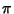be a Plane intersecting a right circular Cone with vertex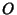in the curve. Call the Spheres Tangent to the Cone and the Planeand, and the Circles on which the Spheres are Tangent to the Coneand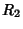. Pick a line along the Cone which intersectsat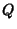,at, andat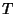. Call the points on the Plane where the Circles are Tangentand. Because intersecting tangents have the same length,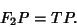Therefore,which is a constant independent of, sois an Ellipse with.

References

Honsberger, R. Kepler's Conics.'' Ch. 9 in Mathematical Plums (Ed. R. Honsberger). Washington, DC: Math. Assoc. Amer., p. 170, 1979.

Honsberger, R. More Mathematical Morsels. Washington, DC: Math. Assoc. Amer., pp. 40-44, 1991.

Ogilvy, C. S. Excursions in Geometry. New York: Dover, pp. 80-81, 1990.

Ogilvy, C. S. Excursions in Mathematics. New York: Dover, pp. 68-69, 1994.